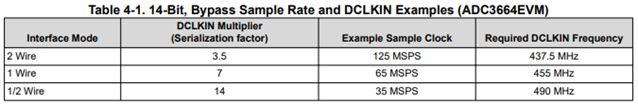If you have a related question, please click the "Ask a related question" button in the top right corner. The newly created question will be automatically linked to this question.

# ADC3660EVM: ADC35XX GUI shows only garbage for DCLKIN and decimation factors >8

Hi,

I tried to calculate the right DCLKIN values with the ADC35XX GUI 1.0 (or ADC3660EVM GUI, as it is also called).

The calculated DCLKIN values seem to be right for BYP or decimation factors up to 8.

For decimation factors of 16 and 32, only garbage numbers appear as results for DCLKIN (MHz) in the dialog window:Is there a simple formula for calculating the DCLKIN frequency by myself, i.e. without using the GUI?

• Christian,

The DCLK relationship changes based on the mode (1/2w, 1w, 2w). The ratio will be the same between bypass and decimation modes. For decimation modes, you can calculate the DCLK = (Sample CLK / decimation rate * ratio)

Thanks, Chase

• Well, Chase, surely you are right, but the AD35XX_GUI seems to calculate the values in a way much different from your equation!

I analyzed the output some more:

With Fs=65MHz and 1/2w, and complex decimation, the ADC35XX_GUI puts out the following DCLKIN values for

BYP, DF=2, DF=4, DF=8, DF=16:

2080 MHz, 520 MHz, 130 MHz, 8,125 MHz, 0,0317 MHz,

With Fs=65MHz and 1w, and complex decimation, the ADC35XX_GUI puts out the following DCLKIN values for

BYP, DF=2, DF=4, DF=8, DF=16:

1040 MHz, 260 MHz, 65 MHz, 4,0625 MHz, 0,01568 MHz

With Fs=65MHz and 2w, and complex decimation, the ADC35XX_GUI puts out the following DCLKIN values for

BYP, DF=2, DF=4, DF=8, DF=16:

520 MHz, 130 MHz, 32 MHz, 2,03 MHz, 0,0079 MHz

The DCLKIN values calculated by the AD35XX_GUI are proportional to DCLK = (Sample CLK / (2**decimation rate) * ratio)

This seems to be a bug for me (or a heavy misunderstanding of the data sheet by me)!?

• Yes, the GUI is incorrect with the decimation DCLK calculations. We've requested this to be corrected on the next version of this software. I'm not sure of a date for when this is ready. I'll check with the expert for this part.

• This table shows the ratios for each of the modes.